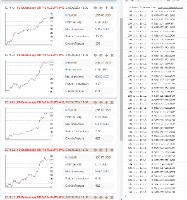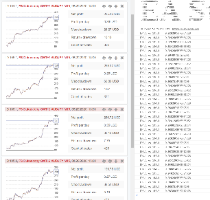# forex software

Create and Test Forex Strategies

forex software

Forex Forum

Forex Software, Forex Strategies, Expert Advisors Generator

# Correlation calculations correct?

Forex Forum → Correlation calculations correct?

Pages 1

## Posts: 5

#### Topic: Correlation calculations correct?

Hi Miroslav,

What is the correlation formula used to calculate correlation between strategies?

EA Studio seems to think that the above strategies are correlated. The correlated setting is set to .98 meaning the strategies should basically be identical but a quick visual inspection of the equity curves indicates otherwise.

Furthermore if I remove strategy 7.3 it removes the correlation for for 4.1, 7.1 and 7.2 indicating that those strategies are correlated to 7.3, but not each other. This leaves a total of 5 strategies.

However if I let EA Studio handle the removal of correlated strategies automatically, it deletes the 4 "correlated" strategies leaving me with a total of 2 strategies.

Why does EA Studio think the 4 strategies are correlated?
What are the 4 strategies correlated to?
Why does EA Studio remove the 4 strategies instead of just removing the 7.3, leaving me with more strategies. If the strategies are in my collection it means they have met my acceptance criteria. And if they met my acceptance criteria I want more of them, not less.

Need help managing a portfolio of hundreds of trading strategies? Check out Strategy Samurai

#### Re: Correlation calculations correct?

The correlated setting is set to .98 meaning the strategies should basically be identical but a quick visual inspection of the equity curves indicates otherwise.

It is a matter of perception and settings.

> Why does EA Studio think the 4 strategies are correlated?
Because the Correlation calculations show a Correlation Coefficient equal to or higher than 0.98. Please see more details here: Pearson correlation coefficient.

The formula is pretty simple and well checked. You may check it also ( the code is below). There aList and bList are the balance curves of two strategies.

> What are the 4 strategies correlated to?

EA Studio checks each strategy against all the rest in the Collection.

> Why does EA Studio remove the 4 strategies instead of just removing the 7.3,

Because EA Studio leaves the strategy with the highest goal ( according to Sort collection by ) form the correlated couples.

> If the strategies are in my collection it means they have met my acceptance criteria. And if they met my acceptance criteria I want more of them, not less.

Excellent! You already figured it out. When you switch the automatic correlation resolution, EA Studio does not remove strategies.

``````    private getCorrelation(aList: number[], bList: number[]): number {
const n: number = Math.min(aList.length, bList.length);
const a1: number = aList;
const b1: number = bList;

let sumX: number = 0;
let sumY: number = 0;
let sumXX: number = 0;
let sumYY: number = 0;
let sumXY: number = 0;

for (let i: number = 1; i < n; i++) {
const x: number = aList[i] - a1;
const y: number = bList[i] - b1;
sumX += x;
sumY += y;
sumXX += x * x;
sumYY += y * y;
sumXY += x * y;
}

const c: number = n - 1;
const covariation: number = sumXY / c - sumX * sumY / c / c;
const aSigma: number = Math.sqrt(sumXX / c - sumX * sumX / c / c);
const bSigma: number = Math.sqrt(sumYY / c - sumY * sumY / c / c);
const correlation: number = covariation / aSigma / bSigma;

return correlation;
}``````

#### Re: Correlation calculations correct?

I decided to check the calculations again (also with an alternative formula).

The calculated Correlation coefficients are shown on the right (my strategies ID numbers are different than yours).Alternative formula (code below):..

I noticed that there actually 3 distinct strategies - those with different major ID number: 1.1, 4.1, and 7.1

Strategies with IDs 7.2 and 7.3 are modified versions of 7.1 with the same trading rules but different numbers.

I don't think I can add more to this topic.

``````    private getCorrelation(aList: number[], bList: number[]) {
const n: number = Math.min(aList.length, bList.length);
const a1: number = aList;
const b1: number = bList;

if (n === 0) {
return 0;
}

let meanX: number = 0;
let meanY: number = 0;
for (let i = 0; i < n; i++) {
meanX += (aList[i] - a1) / n
meanY += (bList[i] - b1) / n
}

let cov: number  = 0;
let den1: number = 0;
let den2: number = 0;

for (let i = 0; i < n; i++) {
const dx: number = (aList[i] - a1) - meanX;
const dy: number = (bList[i] - a1) - meanY;
cov  += dx * dy
den1 += dx * dx
den2 += dy * dy
}

const den = Math.sqrt(den1) * Math.sqrt(den2);

return den === 0 ? 0 : cov / den;
}``````

#### Re: Correlation calculations correct?

Thanks Miroslav.

The alternative calculations looks very similar to the original.

Are there more pages to your correlation calculations? I ask because 6 strategies with a correlation matrix (to each other) should produce 30 unique correlations statistics, (6^6) - 6, but I can't see the rest of the calculations.Excellent! You already figured it out. When you switch the automatic correlation resolution, EA Studio does not remove strategies.

What I'm trying to say is, if the strategies are in my collection that means that they are "good enough". They met my acceptance criteria, regardless of how I decide to sort them in the Collection.

At the end, after correlation analysis, I would much rather have 5 uncorrelated strategies (4 if removing the other correlated strategy) than 2, regardless of the top "Sort collection by" metric because by virtue of being in the collection they are already deemed acceptable.

Is this possible to add a setting to the Correlation Analysis settings where the user can decide what strategies get deleted? Either A) all correlations are deleted excluding the one with the highest Sort by value (current behaviour) or B) the strategy with the highest number or correlations is deleted (if only 1 correlation then the strategy with the highest Sort by value is kept).

An example of how it would look.

A) Strategies 7.1 and 1.1 are kept.B) Strategies 7.3 and 1.1 or 1.2 (depending on the Sort by value) are discarded. We will discard 1.1 in this example.I could perform the correlation analysis myself but EA Studio doesn't provide a correlation matrix. The only way to perform it manually is to save the collection, then randomly start deleting strategies and seeing which one results in less correlations and ultimately the most strategies at the end, Each time re-importing the original collection (6 in this case) and repeating.

-----

What are the list of values of aList and bList? Are they arrays of trade balances or daily balances?

Need help managing a portfolio of hundreds of trading strategies? Check out Strategy Samurai

#### Re: Correlation calculations correct?

I could perform the correlation analysis myself

Yes, you can. The exported Collection file contains a summary of the Balance and Equity charts (compressed to 360 values ( don't remember exactly) and rounded).

You can manage the Collections with your own tools.

Pages 1